# 怎么通过PHP实现给定年月日计算总天数

+关注继续查看

function GetMaxDay($year,$month){
$time = strtotime("{$year}-{$month}"); //取得一个日期的 Unix 时间戳;$date=date("t",$time); return$date;
}

echo GetMaxDay(2000,2);
1.png

for($i=1;$i<$month;$i++){
$sum += GetMaxDay($year,$i); } 测试一下，计算2000年3月之前的所有天数（31+29=60），看看是不是60天： 3.png OK，功能实现了！ 最后将获取的天数和$sum和指定$month的指定日$day的天数相加即可。我们来看看完整代码：

<?php
$year=2000;$month=3;
$day=5; //求一个月的最大天数 function GetMaxDay($year,$month){$time = strtotime("{$year}-{$month}"); //取得一个日期的 Unix 时间戳;
$date=date("t",$time);
return $date; } //求总天数 //求之前几个月的天数$sum=0;
for($i=1;$i<$month;$i++){
$sum += GetMaxDay($year,$i); }$sum = $sum +$day; //加上本月的天数，就是总天数
//求总天数
echo "截止 $year 年$month 月 $day 日， 一共有$sum 天 ";
?>

4.png

<?php
//求一个月的最大天数
function GetMaxDay($year,$month){
$time = strtotime("{$year}-{$month}"); //取得一个日期的 Unix 时间戳;$date=date("t",$time); return$date;
}
//求总天数
function GetDays($year,$month,$day){ //求之前几个月的天数$sum=0;
for($i=1;$i<$month;$i++){
$sum += GetMaxDay($year,$i); }$sum = $sum +$day; //加上本月的天数，就是总天数
//求总天数
echo "截止 $year 年$month 月 $day 日， 一共有$sum 天
";
}
?>

GetDays(2000,3,5);
GetDays(2001,3,5);
GetDays(2001,2,5);

3.png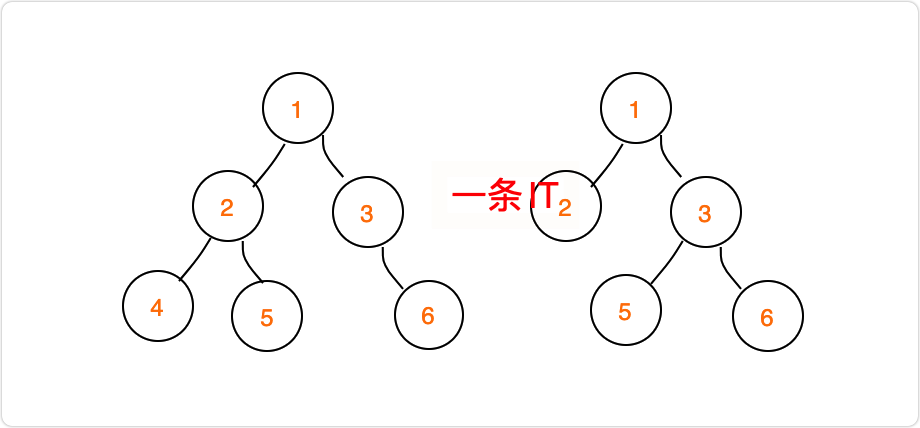【leetcode刷题】29.合并二叉树——Java版
⭐欢迎订阅《leetcode》专栏，每日一题，每天进步⭐ 递归就完事了。。 大一菜鸡艰难刷题中。。 ——leetcode此题热评
3 03 0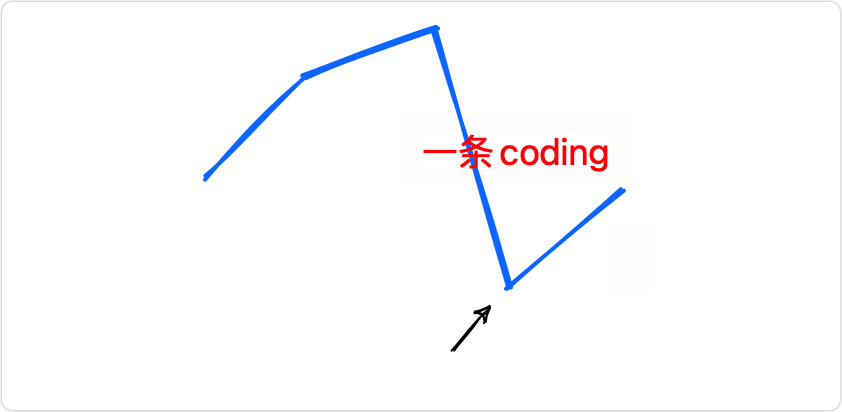【leetcode刷题】31.旋转数组的最小数字——Java版
⭐欢迎订阅《leetcode》专栏，每日一题，每天进步⭐ 什么鬼题目呀，管你旋不旋转，直接输出最小值不就完了。。。 ——leetcode此题热评
4 0【leetcode刷题】32.二进制中1的个数——Java版
⭐欢迎订阅《leetcode》专栏，每日一题，每天进步⭐ 把一个整数减去1，再和原整数做与运算，会把该整数最右边一个1变成0.那么一个整数的二进制有多少个1，就可以进行多少次这样的操作。 ——leetcode此题热评
3 0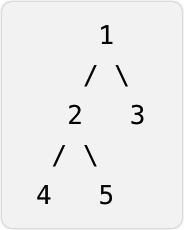【leetcode刷题】27.二叉树的直径——Java版
⭐欢迎订阅《leetcode》专栏，每日一题，每天进步⭐ 可以将二叉树的直径转换为：二叉树的每个节点的左右子树的高度和的最大值。 ——leetcode此题热评
4 0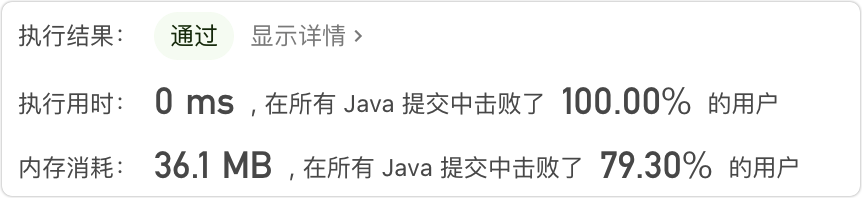【leetcode刷题】28.替换空格——Java版
⭐欢迎订阅《leetcode》专栏，每日一题，每天进步⭐ return s.replace(" ","%20"); ——leetcode此题热评
3 0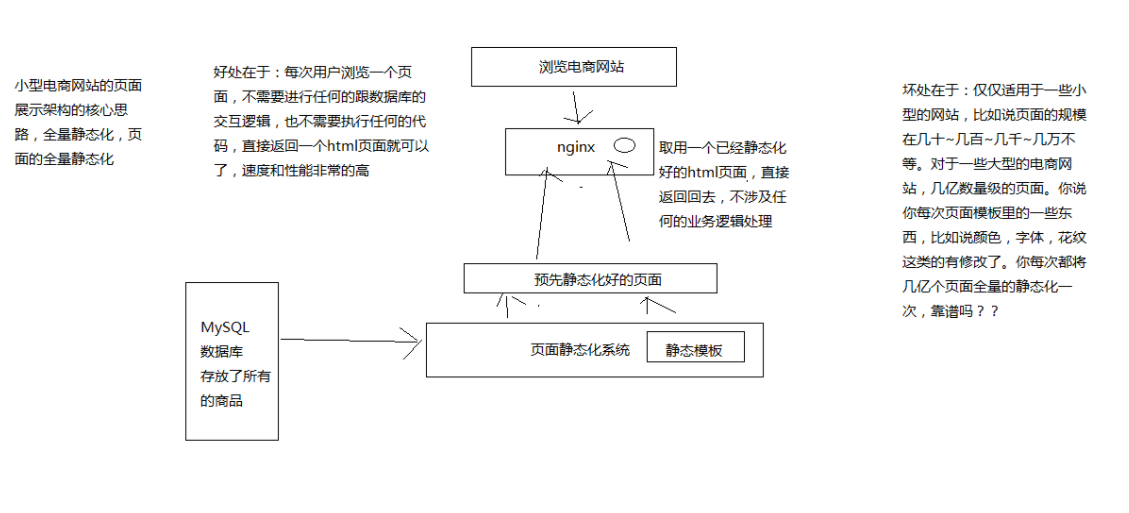4 04 03 0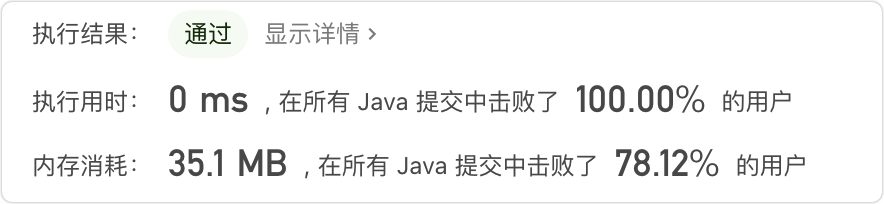【leetcode刷题】30.青蛙跳台阶——Java版
⭐欢迎订阅《leetcode》专栏，每日一题，每天进步⭐ 0级台阶还有1种跳法？这是要在原地来个托马斯旋转吗？ ——leetcode此题热评
3 0
507

0

《SaaS模式云原生数据仓库应用场景实践》

《看见新力量：二》电子书